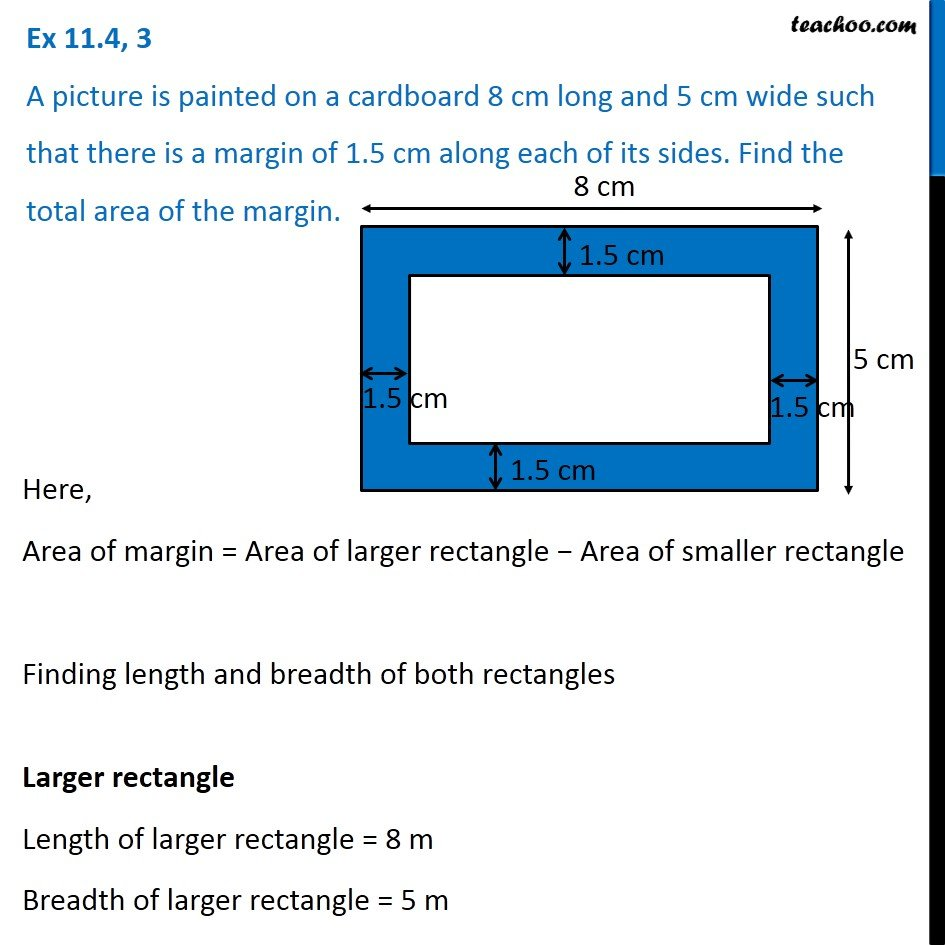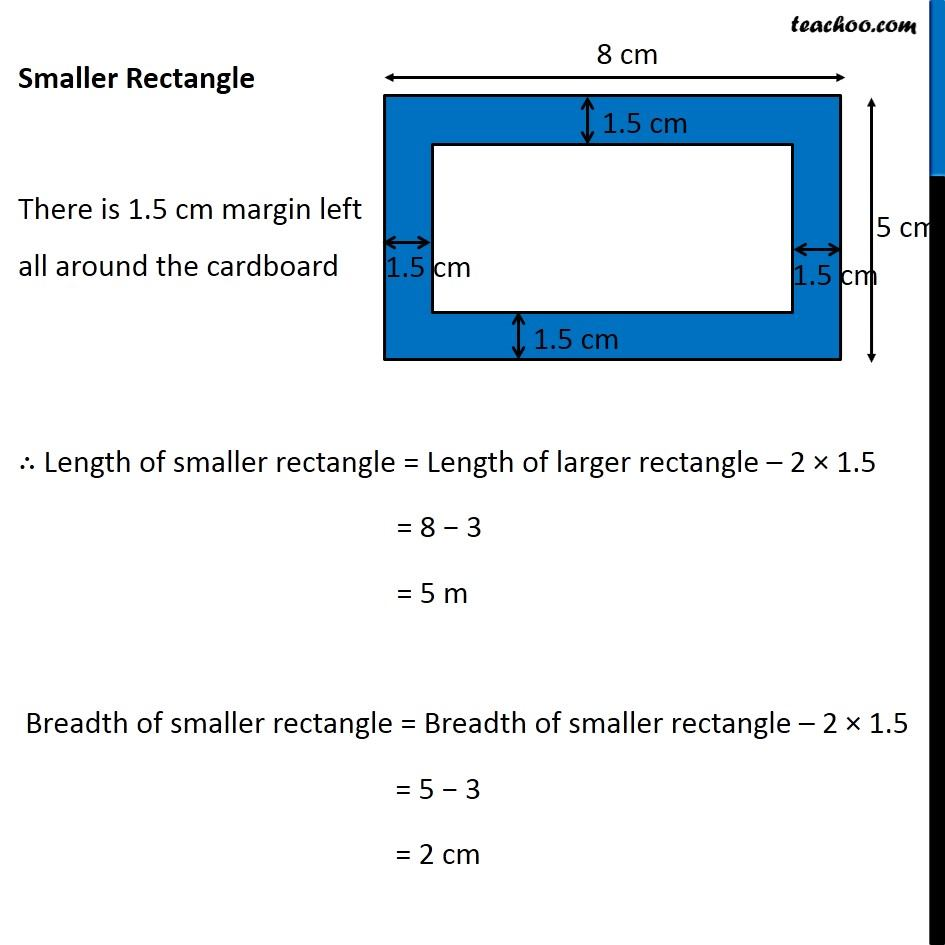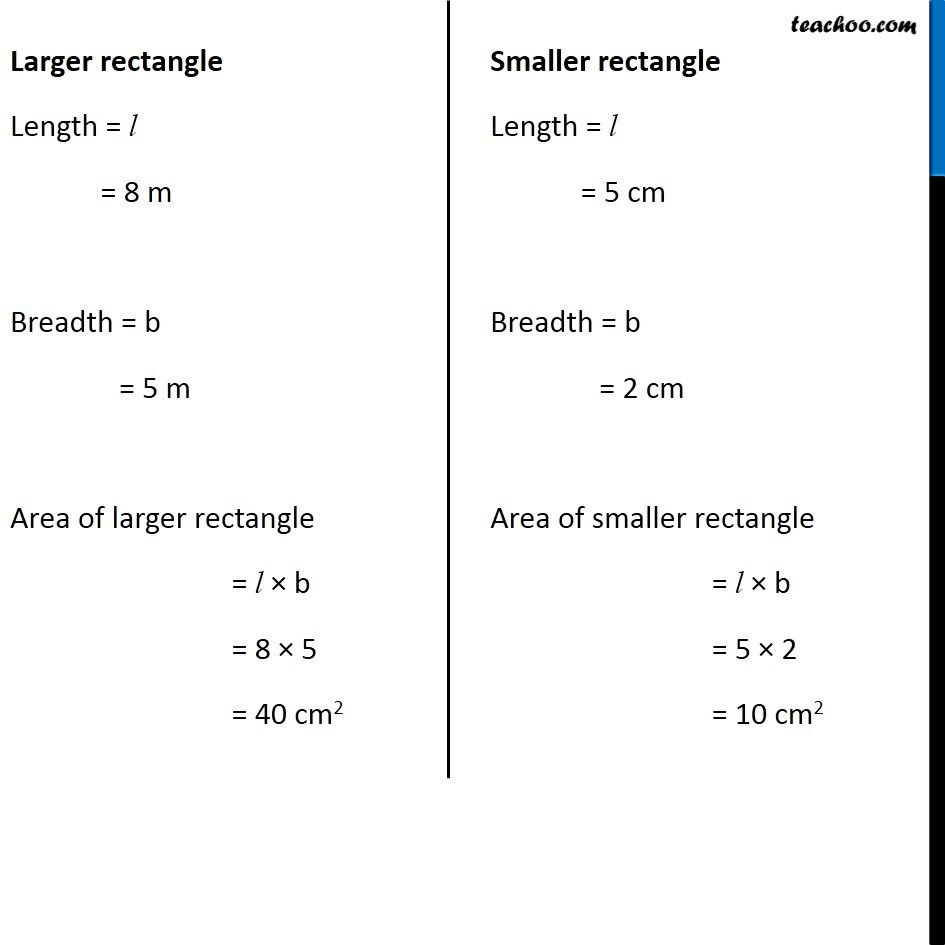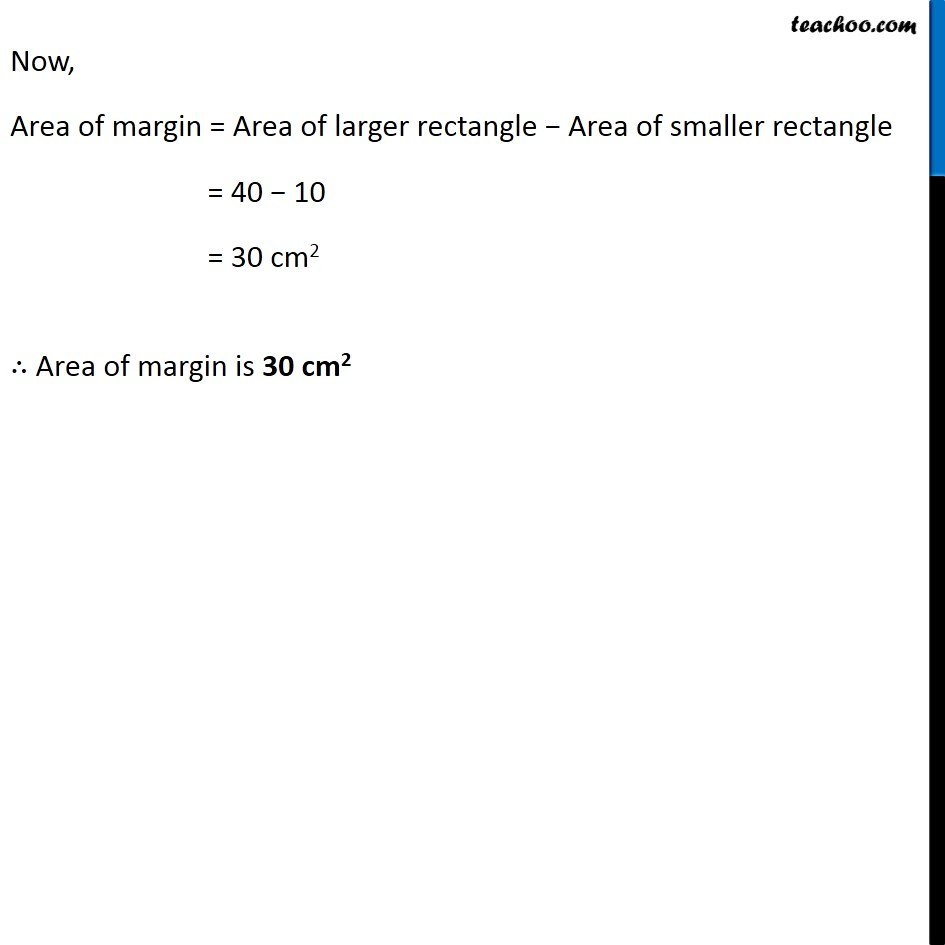1. Chapter 11 Class 7 Perimeter and Area
2. Concept wise
3. Finding Area of rectangle with path outside/inside

Transcript

Ex 11.4, 3 A picture is painted on a cardboard 8 cm long and 5 cm wide such that there is a margin of 1.5 cm along each of its sides. Find the total area of the margin. Here, Area of margin = Area of larger rectangle − Area of smaller rectangle Finding length and breadth of both rectangles Larger rectangle Length of larger rectangle = 8 m Breadth of larger rectangle = 5 m Smaller Rectangle There is 1.5 cm margin left all around the cardboard ∴ Length of smaller rectangle = Length of larger rectangle – 2 × 1.5 = 8 − 3 = 5 m Breadth of smaller rectangle = Breadth of smaller rectangle – 2 × 1.5 = 5 − 3 = 2 cm Larger rectangle Length = l = 8 m Breadth = b = 5 m Area of larger rectangle = l × b = 8 × 5 = 40 cm2 Smaller rectangle Length = l = 5 cm Breadth = b = 2 cm Area of smaller rectangle = l × b = 5 × 2 = 10 cm2 Now, Area of margin = Area of larger rectangle − Area of smaller rectangle = 40 − 10 = 30 cm2 ∴ Area of margin is 30 cm2

Finding Area of rectangle with path outside/inside

About the AuthorDavneet Singh
Davneet Singh is a graduate from Indian Institute of Technology, Kanpur. He has been teaching from the past 10 years. He provides courses for Maths and Science at Teachoo.